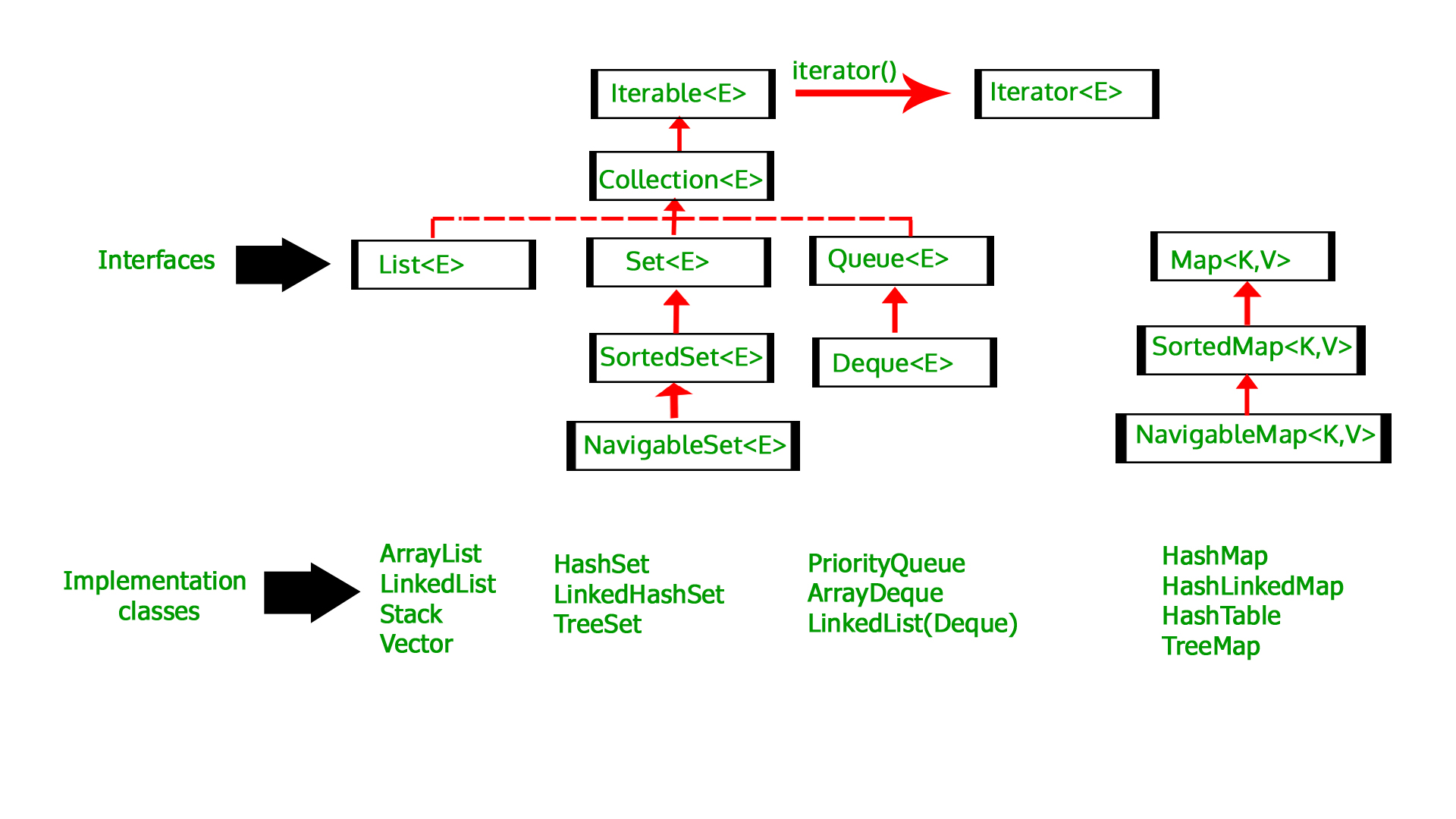# Initialize an ArrayList in Java

ArrayList is a part of collection framework and is present in java.util package. It provides us dynamic arrays in Java. Though, it may be slower than standard arrays but can be helpful in programs where lots of manipulation in the array is needed.

• ArrayList inherits AbstractList class and implements List interface.
• ArrayList is initialized by a size, however the size can increase if collection grows or shrink if objects are removed from the collection.
• Java ArrayList allows us to randomly access the list.
• ArrayList can not be used for primitive types, like int, char, etc. We need a wrapper class for such cases (see this for details).
• ArrayList in Java can be seen as similar to vector in C++.Below are the various methods to initialize an ArrayList in Java:

Syntax:

```ArrayList<Type> str = new ArrayList<Type>();
```

Examples:

 `// Java code to illustrate initialization ` `// of ArrayList using add() method ` ` `  `import` `java.util.*; ` ` `  `public` `class` `GFG { ` `    ``public` `static` `void` `main(String args[]) ` `    ``{ ` ` `  `        ``// create a ArrayList String type ` `        ``ArrayList gfg = ``new` `ArrayList(); ` ` `  `        ``// Initialize an ArrayList with add() ` `        ``gfg.add(``"Geeks"``); ` `        ``gfg.add(``"for"``); ` `        ``gfg.add(``"Geeks"``); ` ` `  `        ``// print ArrayList ` `        ``System.out.println(``"ArrayList : "` `+ gfg); ` `    ``} ` `} `

Output:

```ArrayList : [Geeks, for, Geeks]
```

Examples: Using shorthand version of this method

 `// Java code to illustrate initialization ` `// of ArrayList using add() method ` ` `  `import` `java.util.*; ` ` `  `public` `class` `GFG { ` `    ``public` `static` `void` `main(String args[]) ` `    ``{ ` ` `  `        ``// create a ArrayList String type ` `        ``// and Initialize an ArrayList with add() ` `        ``ArrayList gfg = ``new` `ArrayList() { ` `            ``{ ` `                ``add(``"Geeks"``); ` `                ``add(``"for"``); ` `                ``add(``"Geeks"``); ` `            ``} ` `        ``}; ` ` `  `        ``// print ArrayList ` `        ``System.out.println(``"ArrayList : "` `+ gfg); ` `    ``} ` `} `

Output:

```ArrayList : [Geeks, for, Geeks]
```
2. ### Initialization using asList()

Syntax:

```ArrayList<Type> obj = new ArrayList<Type>(
Arrays.asList(Obj A, Obj B, Obj C, ....so on));
```

Examples:

 `// Java code to illustrate initialization ` `// of ArrayList using asList method ` ` `  `import` `java.util.*; ` ` `  `public` `class` `GFG { ` `    ``public` `static` `void` `main(String args[]) ` `    ``{ ` ` `  `        ``// create a ArrayList String type ` `        ``// and Initialize an ArrayList with asList() ` `        ``ArrayList gfg = ``new` `ArrayList( ` `            ``Arrays.asList(``"Geeks"``, ` `                          ``"for"``, ` `                          ``"Geeks"``)); ` ` `  `        ``// print ArrayList ` `        ``System.out.println(``"ArrayList : "` `+ gfg); ` `    ``} ` `} `

Output:

```ArrayList : [Geeks, for, Geeks]
```
3. ### Initialization using List.of() method

Syntax:

```List<Type> obj = new ArrayList<>(
List.of(Obj A, Obj B, Obj C, ....so on));
```

Examples:

 `// Java code to illustrate initialization ` `// of ArrayList using List.of() method ` ` `  `import` `java.util.*; ` ` `  `public` `class` `GFG { ` `    ``public` `static` `void` `main(String args[]) ` `    ``{ ` ` `  `        ``// create a ArrayList String type ` `        ``// and Initialize an ArrayList with List.of() ` `        ``List gfg = ``new` `ArrayList<>( ` `            ``List.of(``"Geeks"``, ` `                    ``"for"``, ` `                    ``"Geeks"``)); ` ` `  `        ``// print ArrayList ` `        ``System.out.println(``"ArrayList : "` `+ gfg); ` `    ``} ` `} `

Output:

```ArrayList : [Geeks, for, Geeks]
```
4. ### Initialization using another Collection

Syntax:

```List gfg = new ArrayList<>(collection);
```

Examples:

 `// Java code to illustrate initialization ` `// of ArrayList using another collection ` ` `  `import` `java.util.*; ` ` `  `public` `class` `GFG { ` `    ``public` `static` `void` `main(String args[]) ` `    ``{ ` ` `  `        ``// create another collection ` `        ``List arr = ``new` `ArrayList<>(); ` `        ``arr.add(``1``); ` `        ``arr.add(``2``); ` `        ``arr.add(``3``); ` `        ``arr.add(``4``); ` `        ``arr.add(``5``); ` ` `  `        ``// create a ArrayList Integer type ` `        ``// and Initialize an ArrayList with arr ` `        ``List gfg = ``new` `ArrayList(arr); ` ` `  `        ``// print ArrayList ` `        ``System.out.println(``"ArrayList : "` `+ gfg); ` `    ``} ` `} `

Output:

```ArrayList : [1, 2, 3, 4, 5]
```
5. Attention reader! Don’t stop learning now. Get hold of all the important Java and Collections concepts with the Fundamentals of Java and Java Collections Course at a student-friendly price and become industry ready.

My Personal Notes arrow_drop_upCheck out this Author's contributed articles.

If you like GeeksforGeeks and would like to contribute, you can also write an article using contribute.geeksforgeeks.org or mail your article to contribute@geeksforgeeks.org. See your article appearing on the GeeksforGeeks main page and help other Geeks.

Please Improve this article if you find anything incorrect by clicking on the "Improve Article" button below.

Article Tags :
Practice Tags :

11

Please write to us at contribute@geeksforgeeks.org to report any issue with the above content.